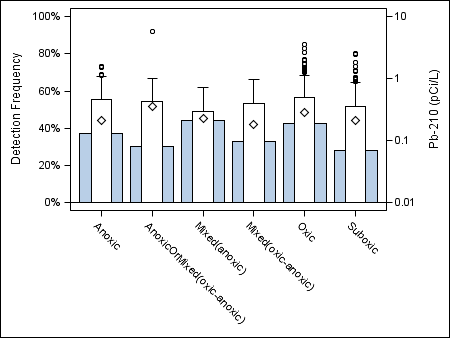## How to combine categorical and distributional plots

I'd like to overlay boxplots (y2axis) on bar plots (yaxis). These 2 plot types are not compatible in sgplot. What are my options?

1 ACCEPTED SOLUTION

Accepted Solutions

## Re: How to combine categorical and distributional plots

Can you show an example of what you're trying to accomplish?

You can look into custom plots using GTL and note that you can use TMPLOUT option on PROC SGPLOT to generate the base statements for you to get started.

http://documentation.sas.com/?cdcId=pgmmvacdc&cdcVersion=9.4&docsetId=grstatproc&docsetTarget=p073bl...

@pstack wrote:

I'd like to overlay boxplots (y2axis) on bar plots (yaxis). These 2 plot types are not compatible in sgplot. What are my options?

8 REPLIES 8

## Re: How to combine categorical and distributional plots

Can you show an example of what you're trying to accomplish?

You can look into custom plots using GTL and note that you can use TMPLOUT option on PROC SGPLOT to generate the base statements for you to get started.

http://documentation.sas.com/?cdcId=pgmmvacdc&cdcVersion=9.4&docsetId=grstatproc&docsetTarget=p073bl...

@pstack wrote:

I'd like to overlay boxplots (y2axis) on bar plots (yaxis). These 2 plot types are not compatible in sgplot. What are my options?

## Re: How to combine categorical and distributional plots

Thank you for pointing me in the right direction. I had never used GTL but it was the solution. In short order I made the plot shown below which is what I wanted. This particular version of the plot is not very enlightening but in some circumstances it nicely displays that detection frequencies and concentration distributions vary significantly.DanH_sas
SAS Super FREQ

## Re: How to combine categorical and distributional plots

This can be done using sgplot, but you must use a BARPARM instead of a BAR. This also means that you will need to pre-summarize the bar statistics. Here are a couple of examples:

``````proc summary data=sashelp.class nway;
class age;
var weight;
output out=meanwgt mean=meanwgt;
run;

data merged;
merge meanwgt(rename=(age=age1)) sashelp.class;
run;

proc sgplot data=merged;
vbarparm category=age1 response=meanwgt;
vbox height / category=age y2axis fillattrs=(color=red);
run;

proc sgplot data=merged;
vbarparm category=age1 response=meanwgt / barwidth=0.4 discreteoffset=-0.2;
vbox height / category=age y2axis fillattrs=(color=red) boxwidth=0.4 discreteoffset=0.2;
run;
``````

Hope this helps!

Dan

## Re: How to combine categorical and distributional plots

Thanks Dan. I copied and pasted your example code into SAS but still got the "incompatible plot types" error messages. Below is a snippet from the log showing that the data was read in...but the plots could not be made:

NOTE: There were 6 observations read from the data set WORK.MEANWGT.

NOTE: There were 19 observations read from the data set SASHELP.CLASS.

NOTE: The data set WORK.MERGED has 19 observations and 9 variables.

NOTE: DATA statement used (Total process time):

real time 0.01 seconds

cpu time 0.00 seconds

10

11 proc sgplot data=merged;

12 vbarparm category=age1 response=meanwgt;

13 vbox height / category=age y2axis fillattrs=(color=red);

14 run;

NOTE: Writing HTML Body file: sashtml.htm

ERROR: Attempting to overlay incompatible plot or chart types.

NOTE: The SAS System stopped processing this step because of errors.

NOTE: PROCEDURE SGPLOT used (Total process time):

real time 0.17 seconds

cpu time 0.10 seconds

15

16 proc sgplot data=merged;

17 vbarparm category=age1 response=meanwgt / barwidth=0.4 discreteoffset=-0.2;

18 vbox height / category=age y2axis fillattrs=(color=red) boxwidth=0.4 discreteoffset=0.2;

19 run;

ERROR: Attempting to overlay incompatible plot or chart types.

NOTE: The SAS System stopped processing this step because of errors.

NOTE: PROCEDURE SGPLOT used (Total process time):

real time 0.00 seconds

cpu time 0.00 secondsDanH_sas
SAS Super FREQ

## Re: How to combine categorical and distributional plots

What version of SAS are you using?DanH_sas
SAS Super FREQ

## Re: How to combine categorical and distributional plots

Support for this type of overlay was added starting with 9.4, maintenance 1.

SAS 9.4

## Re: How to combine categorical and distributional plots

That must be it. I don't see anything about maintenance level:

SAS 9.4 TS Level 1M0

X64_7PRO platform

I have a work around for this using Proc Template and assigning and sorting the x-axis with a numeric thanks to previous responses.

Thanks for the help. -Paul

Discussion stats
• 8 replies
• 1034 views
• 1 like
• 3 in conversation